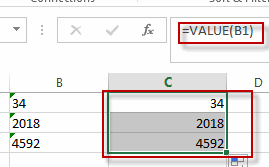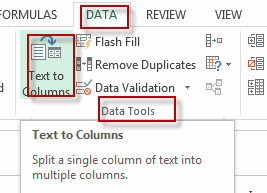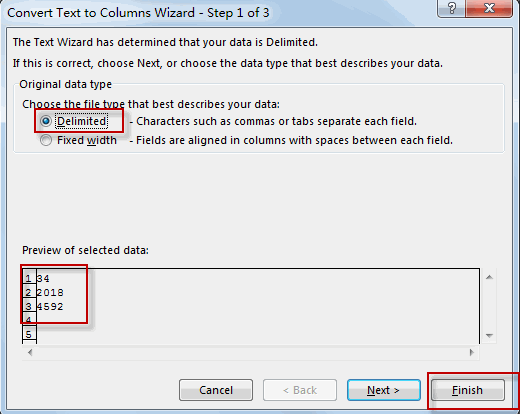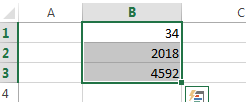Remove Apostrophe or Text Indicator

This post will guide you how to remove the apostrophe (‘) from the text values in your worksheet in excel. How do I remove text indicator from a text string in a cell in excel. How to use an excel formula to remove text indicator quickly. How to remove leading Apostrophe from a text value in a cell.

Remove Apostrophe with Excel Formula

If you want to remove the text indicator (‘) or the leading Apostrophe from a text string in one cell, you can create a formula based on the VALUE function.Just like this:

=VALUE(B1)

You just need to type this formula into the formula box of the cell C1, then press Enter key in your keyboard. And drag the AutoFill Handler over other cells to apply this formula.

Let’s see the result:Remove Apostrophe with Text to Columns Function

You can also use the Text to Columns function to remove the text indicator from text values in one cell, just do the following steps:

#1 select the cells that you want to remove the Apostrophe or text indicator, such as: B1:B3

#2 go to DATA tab, click Text to Columns command under Data Tools group. And the Convert Text to Columns Wizard dialog will appear.#3 select the Delimited radio button, and then click Finish button.#4 you will see the text indicators have been removed.Related Functions

• Excel VALUE function
The Excel VALUE function converts a text value that represents a number to a numeric value.The syntax of the VALUE function is as below:= VALUE  (text)…
Related Posts

How to Use 3D SUM Multiple Worksheets

To sum a range of numbers is straightforward for most Excel users, but do you know how to establish a 3D reference to total the same range of numerous sheets. In this post, I will present the steps for this ...

Extract all the matches with helper Column

With Excel's powerful functions IF, INDEX, and MATCH, we can find exactly what you're looking for with a few clicks of the mouse. This step-by-step tutorial will show how easy it is to extract data using these tools and more! ...

If Cell is This Value or That Value

IF function is frequently used in Excel worksheet to return you expect “true value” or “false value” based on the result of logical test. If you want to see if a cell is A or B, and if one of ...

If Value is Greater Than A Certain Value

IF function is frequently used in Excel worksheet to return you expect “true value” or “false value” based on the logical test result. If you want to see if a value in one cell is greater than a specific value, ...

If Cell is Not Blank

IF function is frequently used in Excel worksheet to return you expect “true value” or “false value” based on the result of created logical test. If you want to see if a cell is blank or not, and leave some ...

If Cell is Blank

IF function is frequently used in Excel worksheet to return you expect “true value” or “false value” based on the result of created logical test. If you want to see if a cell is blank or not, and leave some ...

If Cell Equals Certain Text String

IF function is frequently used in Excel worksheet to return you expect “true value” or “false value” based on the result of created logical test. If you want to see if cell equals a certain text string like “Win”, you ...

If Cell Contains Either Text1 or Text2

IF function is frequently used in Excel worksheet to return “true value” or “false value” based on the logical test result. If you want to see if cell contains certain substring1 like “abc” or substring2 like “def”, and returns true ...

If Cell Contains Certain Text OR Equals Certain Text

IF cell equals certain text IF function is frequently used in Excel worksheet to return “true value” or “false value” based on the logical test result. If you want to test values to see if they equal certain text like ...

VLOOKUP From Another Sheet Not Working

In the previous post, you should know that how to fix or remove the #N/A error when using VLOOKUP formula to lookup value from another sheet. And this post will show you reasons why your VLOOKUP formula is not working ...

Sidebar